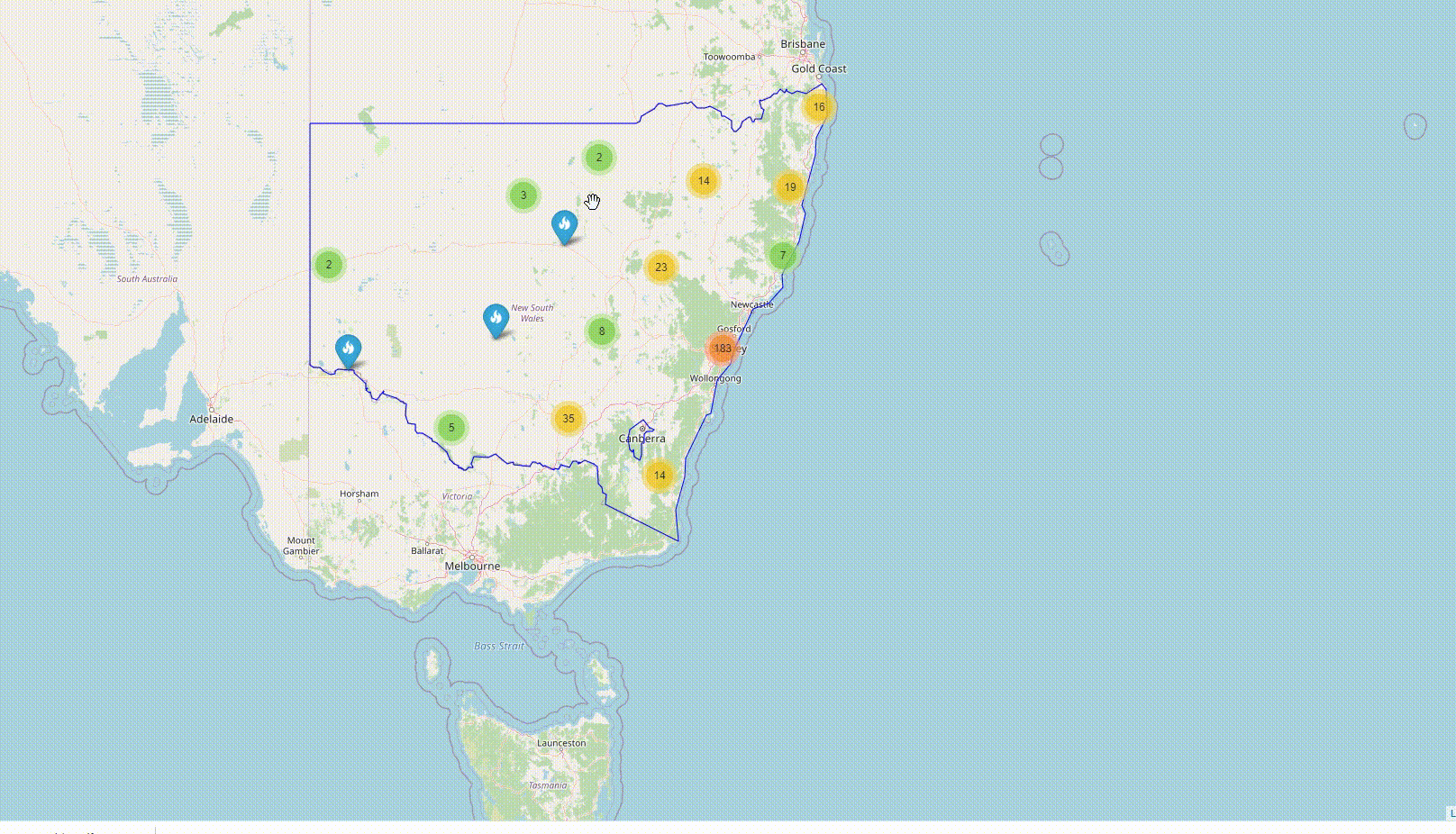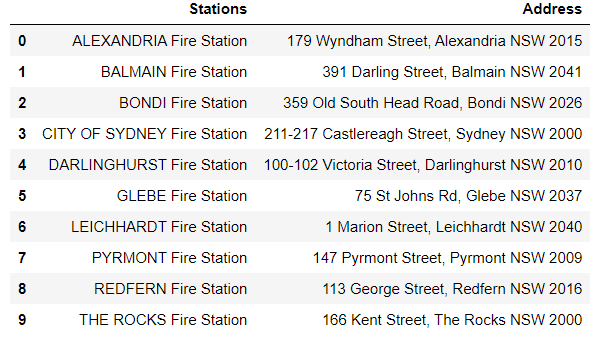# Converting Nested List into a Pandas DataFrameIn this article, I am converting the nested listed into a single list. We will convert the flattened list into a DataFrame. The structure of a nested list looks similar to this: [[list 1],[list 2],[list3], ..…, [list n]].

This is part of the data-preprocessing to generate the HTML map page shown below.In the previous article, I scarped a website using BeautifulSoup, and the data is retrieved in the form of a nested list. In this article, I am converting the nested listed into a single list.

### Import nested list from a text file

You can follow the steps provided in the previous article to generate your nested list or download the nested list from my Github repository. ‘sta.txt’ file contains a nested list of station names, and ‘add.txt’ contains a nested list of corresponding station addresses.

``````# 'sta.txt' contains nested list of stations
content = open("sta.txt", "r")
content.close()

content.close()
``````

A new variable is created from the ‘sta’ to convert the nested list to a single list. The following code is used to flatten the nested list ‘sta’ to a list called ‘all_stations’.

``````# sta is a nested list [[],[],[]]
all_stations = []
for stations in sta:
for station in stations:
all_stations.append(station)
``````

The same process is repeated to convert a nested list ‘add’ to a single list ‘all_address’. The code is given below:

``````# add is a nested list [[],[],[]]
``````

### Converting the lists to a DataFrame

To create a DataFrame, we will first assign the newly created list to pd.DataFrame and assign column name as ‘station’. We will also add a column that contains the station addresses. Both lines of codes are given below.

``````df = pd.DataFrame(all_stations,columns=['Stations'])
``````The next article will extract the information related to the latitude and longitudinal coordinates based on the addresses extracted from the web page and stored in the DataFrame. You can read the next article here: Part 3: Finding latitude and longitude of addresses using GoogleMaps API

GitHub